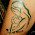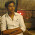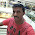VHDL coding tips and tricks: 4 bit Ripple Carry Adder using basic logic gates

## Sunday, March 28, 2010

### 4 bit Ripple Carry Adder using basic logic gates

Here is the code for 4 bit Ripple Carry Adder using basic logic gates such as AND,XOR,OR etc.The module has two 4-bit inputs which has to be added, and one 4-bit output which is the sum of the given numbers.Another output bit indicates whether there is a overflow in the addition,that means whether a carry is generated or not.

--libraries to be used are specified here
library IEEE;
use IEEE.STD_LOGIC_1164.ALL;

--entity declaration with port definitions
entity rc_adder is
port(    num1 : in std_logic_vector(3 downto 0);  --4 bit input 1
num2 :      in std_logic_vector(3 downto 0);  -- 4 bit input 2
sum : out std_logic_vector(3 downto 0);   -- 4 bit sum
carry :  out std_logic   -- carry out.
);
end rc_adder;

--architecture of entity
architecture Behavioral of rc_adder is

--temporary signal declarations(for intermediate carry's).
signal c0,c1,c2,c3 : std_logic := '0';
begin

--first full adder
sum(0) <= num1(0) xor num2(0);  --sum calculation
c0 <= num1(0) and num2(0);          --carry calculation
--second full adder
sum(1) <= num1(1) xor num2(1) xor c0;
c1 <= (num1(1) and num2(1)) or (num1(1) and c0) or (num2(1) and c0);
--third full adder
sum(2) <= num1(2) xor num2(2) xor c1;
c2 <= (num1(2) and num2(2)) or (num1(2) and c1) or (num2(2) and c1);
--fourth(final) full adder
sum(3) <= num1(3) xor num2(3) xor c2;
c3 <= (num1(3) and num2(3)) or (num1(3) and c2) or (num2(3) and c2);
--final carry assignment
carry <= c3;

end Behavioral;

The test bench program used for testing the design is given below:

library IEEE;
use IEEE.STD_LOGIC_1164.ALL;

--this is how entity for your test bench code has to be declared.
entity testbench is
end testbench;

architecture behavior of testbench is
--signal declarations.
signal num1,num2,sum : std_logic_vector(3 downto 0) :=(others => '0');
signal carry :  std_logic:='0';

begin
--entity instantiation
UUT : entity work.rc_adder port map(num1,num2,sum,carry);
--definition of simulation process
tb : process

begin
num1<="0010";  --num1 =2
num2<="1001";  --num2 =9
wait for 2 ns;

num1<="1010";  --num1 =10
num2<="0011";   --num2 =3
wait for 2 ns;

num1<="1000";  --num1 =8
num2<="0101";  --num2 =5
wait for 2 ns;

num1<="1010";  --num1 =10
num2<="0110";  --num2 =6
--more input combinations can be given here.
wait;
end process tb;

end;

The simulated waveform is shown below:

The code was synthesized using XILINX ISE XST . The RTL schematic of the design is shown below:

Note :- Use RTL Viewer to get a closer look on how your design is implemented in hardware.

#### 14 comments:

1.could you please tell me the types of adders ...

i know

carry save adder
carry ripple adder
carry look ahead adder

if any adder besides this .....and also which one is best while we code it in vhdl.....and why..??

asmita

2.hello could you please provide me with the block diagram you used for this coding of carry ripple adder...

thanks in advance

regards
asmita

3.@asmi : Ripple adder gives the worst performance, but it is to implement. So if speed is important I suggest go for carry save or carry look ahead adder. Carry save adder is relatively easy to implement. See this:
http://www.ece.tamu.edu/~sshakkot/courses/ecen248/csa-notes.pdf

Some more adders are:
1)Kogge-Stone adder(the fastest adder)
2)Carry bypass adder.

It is difficult to see which adder is the most useful.One way to find out is implementing all of them.

This is the block diagram I used to code the ripple carry adder here:
http://en.labs.wikimedia.org/wiki/File:4-bit_ripple_carry_adder.svg

4.hello can you please give me vhdl codes for kogge stone adder

5.can any one post carry save adder carry select adder?

6.can anybody gve me the vhdl code for carry save adder

7.i have to impement diff adders and multipliers .plse help me

8.@vipin : thanxx ....

9.can you help me with verilog coding for 54 bit input using carry propagate adder

10.please tell me about the architecture or logic diagram of carry save adder.

11.can any body provide me the vhdl code for carry select adder

12.can u help me to write the code in vhdl ..4 bits alu (4 logical ) nd (8 arithematices) operation

13.can u help me by providing vhdl coding for carry select adder?

14.Can u help me to write code for 32bit unsigned multiplier using carry look ahead adder (vhdl code)